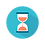From: Bayesian Models for Astrophysical Data, Cambridge Univ. Press

(c) 2017,  Joseph M. Hilbe, Rafael S. de Souza and Emille E. O. Ishida

you are kindly asked to include the complete citation if you used this material in a publication

Code 6.21 Zero-truncated Poisson data

====================================================

set.seed(123579)
nobs <- 1500

x1 <- rbinom(nobs,size=1,0.3)
x2 <- rbinom(nobs,size=1,0.6)
x3 <- runif(nobs)

xb <- 1 + 2*x1 - 3*x2 - 1.5*x3
exb <- exp(xb)

rztp <- function(N, lambda){
p <- runif(N, dpois(0, lambda), 1)
ztp <- qpois(p, lambda)
return(ztp)
}

ztpy <- rztp(nobs, exb)
ztp <- data.frame(ztpy, x1, x2, x3)

====================================================

Code 6.22 Zero-truncated Poisson with zero trick

====================================================

library(MASS)
library(R2jags)

X <- model.matrix(~ x1 + x2 + x3, data = ztp)
K <- ncol(X)

model.data <- list(Y = ztp\$ztpy,
X = X,
K = K,                                                 # number of betas
N = nobs,                                            # sample size
Zeros = rep(0, nobs))

ZTP<-"
model{
for (i in 1:K) {beta[i] ~ dnorm(0, 1e-4)}

# Likelihood with zero trick
C <- 1000
for (i in 1:N) {
Zeros[i] ~ dpois(-Li[i] + C)
Li[i] <- Y[i] * log(mu[i]) - mu[i] -
loggam(Y[i]+1) - log(1-exp(-mu[i]))
log(mu[i]) <- inprod(beta[], X[i,])
}
}"

inits <- function () {
list(beta = rnorm(K, 0, 0.1) )}

params <- c("beta")

ZTP1 <- jags(data = model.data,
inits = inits,
parameters = params,
model = textConnection(ZTP),
n.thin = 1,
n.chains = 3,
n.burnin = 4000,
n.iter = 5000)

print(ZTP1, intervals=c(0.025, 0.975), digits=3)

====================================================Output  on screen:

Inference for Bugs model at "3", fit using jags,

3 chains, each with 5000 iterations (first 4000 discarded)

n.sims = 3000 iterations saved

mu.vect      sd.vect                 2.5%                  97.5%       Rhat         n.eff

beta                     0.985        0.060                0.859                   1.096       1.027         100

beta                     1.996        0.054                1.895                   2.107       1.017         130

beta                    -3.100       0.104                -3.308                 -2.904       1.002       1400

beta                    -1.413       0.081                -1.566                 -1.248       1.009         290

deviance      3002456.898       2.957      3002453.247      3002464.461       1.000             1

For each parameter, n.eff is a crude measure of effective sample size,

and Rhat is the potential scale reduction factor (at convergence, Rhat=1).

DIC info (using the rule, pD = var(deviance)/2)

pD = 4.4 and DIC = 3002461.3

DIC is an estimate of expected predictive error (lower deviance is better).